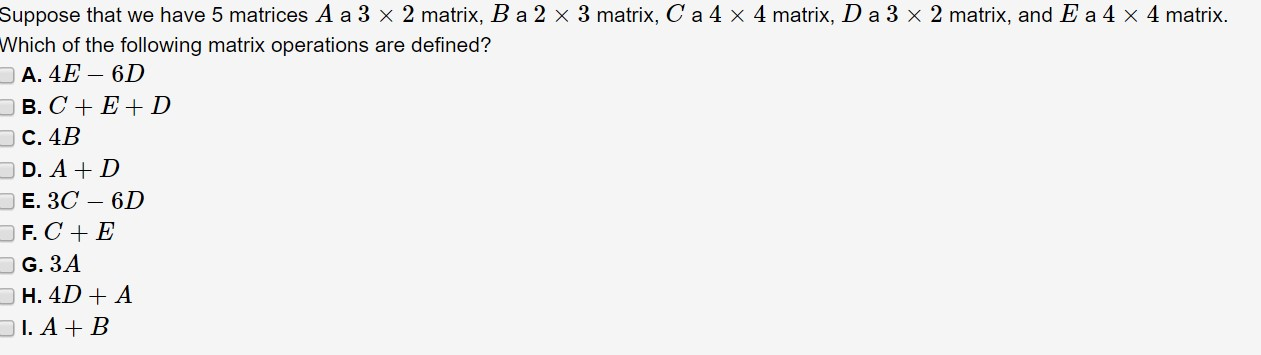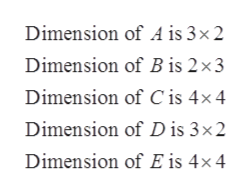# Suppose that we have 5 matrices A a 3 x 2 matrix, B a 2 x 3 matrix, C a 4 x 4 matrix, D a 3 x 2 matrix, and E a 4 x 4 matrix.Which of the following matrix operations are defined?A. 4E- 6DD в. С + Е+DDс. 4BD. A DЕ. ЗС — 6DF. C EG. 3AH. 4D AI. A B

Questionhelp_outlineImage TranscriptioncloseSuppose that we have 5 matrices A a 3 x 2 matrix, B a 2 x 3 matrix, C a 4 x 4 matrix, D a 3 x 2 matrix, and E a 4 x 4 matrix. Which of the following matrix operations are defined? A. 4E- 6D D в. С + Е+D Dс. 4B D. A D Е. ЗС — 6D F. C E G. 3A H. 4D A I. A B fullscreen
check_circleExpert Solution
Step 1

Given dimensions of 5 matrices are,help_outlineImage TranscriptioncloseDimension of A is 3x 2 Dimension of Bis 2x3 Dimension of C is 4x 4 Dimension of D is 3x2 Dimension of E is 4x 4 fullscreen
Step 2

A).Matrix operation 4E - 6D is not defined because dimensions of E and D are not equal.

B).Matrix operation C + E + D is not defined because dimensions of C, E and D are not  equal.

C).Ma...

### Want to see the full answer?

See Solution

#### Want to see this answer and more?

Solutions are written by subject experts who are available 24/7. Questions are typically answered within 1 hour*

See Solution
*Response times may vary by subject and question
Tagged in

### Math